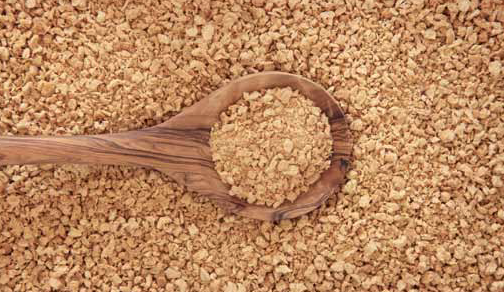## ﻿Mineral

Farm - Home - Lawn & Garden Supplies - Serving Western New York

## Supplements

“OUR” Mineral Supplements:

”OUR” Dry Cow Mineral Supplement (Yellow Tag Mineral)
Mineral - Vitamin - Mix
Phos. =12%
Calcium =Trace
Protein(fr N.P.N.) = 34%
Sulphur = 3.3%
Potassium = 2.7%
Magnesium = 1.65%
Salt = 29%
Znic =.288%
Copper =.025%
Manganese =.120%
Iodine =.004%
Cobalt =.001%
Iron =.027%
Selenium =.0015%
Vitamin A =288,000 I.U./lb
Vitamin D3 = 80,000 I.U./lb
Vitamin E = 1920 I.U./lb

”OUR” One to One (Green Tag Mineral)
Mineral - Vitamin - Mix
Calcium =12%
Phos. =12%
Sulphur = 1.65%
Potassium = 1.35%
Magnesium = .825%
Salt = 20%
Znic =.270%
Copper =.023%
Manganese =.112%
Iodine =.004%
Cobalt =.001%
Iron =.025%
Selenium =.00075%
Vitamin A =194,000 I.U./lb
Vitamin D3 = 97,000 I.U./lb
Vitamin E = 192 I.U./lb

”OUR” B-1360 Cattle Mineral Supplement (White Tag Mineral)
(Medicated)
Aids in control of coccidiosis in cattle weighing up to 800 lbs
Increases rate of gain in cattle

Lasalocid = 1360 gms./ton

Calcium =10%
Phos. = 6%
Sulphur = 1.32%
Potassium = 1.08%
Magnesium = .66%
Salt = 16%
Znic =.144%
Copper =.012%
Manganese =.06%
Iodine =.0024%
Cobalt =.001%
Iron =.014%
Selenium =.00078%
Vitamin A =90,000 I.U./lb
Vitamin D3 = 30,000 I.U./lb
Vitamin E = 600 I.U./lb

”OUR” Big Buck Mineral Supplement
Mineral - Vitamin - Mix
Calcium =12%
Phos. =12%
Sulphur = 1.65%
Potassium = 1.35%
Magnesium = .825%
Salt = 19%
Znic =2700 ppm
Copper = 200 ppm
Manganese =1120 ppm
Iodine = 40 ppm
Cobalt = 10 ppm
Iron = 250 ppm
Selenium = 7.5 ppm
Vitamin A =190 KIU/lb
Vitamin D3 = 97 KIU/lb
Vitamin E = 190 IU/lb